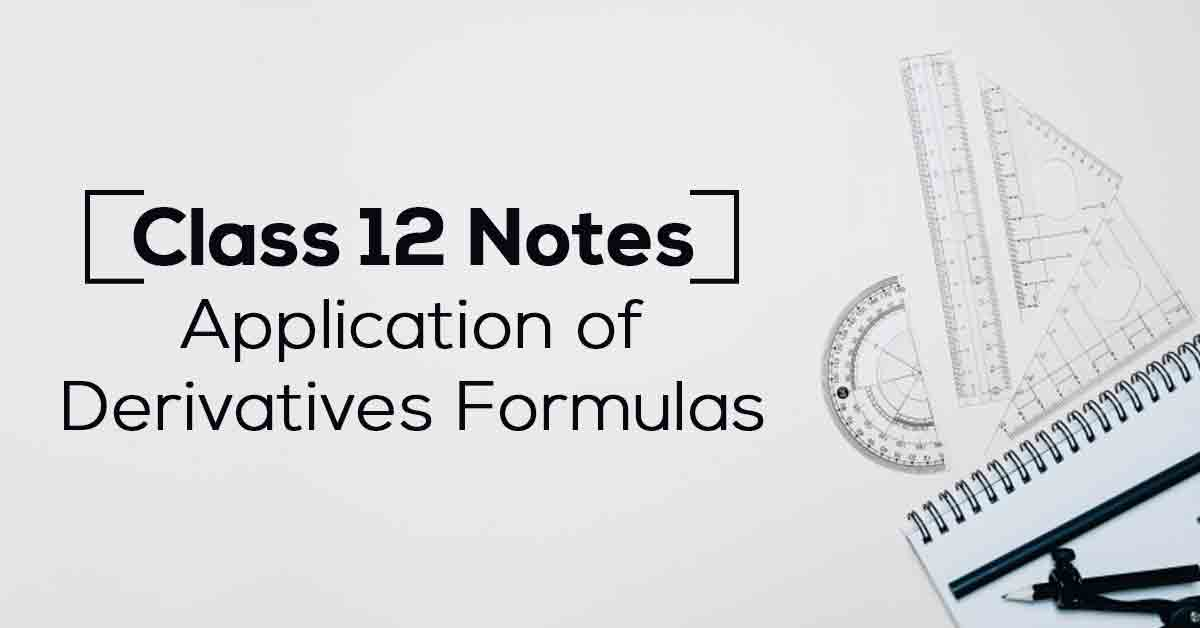Class 12th Math Application Of Derivatives Formulas CBSE 2023

# Application of Derivatives Class 12 Notes# Chapter 6 Application of Derivatives Formulas

The NCERT notes for Class 12 Maths Chapter 6 Application of Derivatives answers all of the questions in the Class 12th Mathematics syllabus for 2022-23. These NCERT notes include a comprehensive set of questions and answers organised at an advanced level of difficulty, giving students ample opportunity to apply their knowledge.

Vidyakul intends to help students understand the basics of the chapter in a fun learning way. Students can easily track their progress on Vidyakul and work accordingly. Read on to know more.

### Points to Remember

Some of the important points to remember from Class 12 Maths Chapter 6 are as follows:

• Application of Derivatives is widely used in engineering, science, economics, and social science, among other fields.

• One of the most important applications of derivatives is to find the best solution to problems. For example, finding the equations of tangent and normal to a curve at a point, or turning points on a function’s graph, will help us locate points where the largest or smallest value (locally) of a function occurs.

• Derivatives can also be used to calculate the intervals at which a function increases or decreases. Finally, it is useful to determine the approximate value of specific quantities.

• The derivatives are used to compute the rate of change, intervals of increasing or decreasing functions, the point at which the tangent is parallel or perpendicular, the approximate value of numbers, and so on.

• The first derivative test is used to find the extremum point of functions by analysing their first derivatives.

• The Second Order Derivative is the derivative of the given function’s first derivative.

• A derivative test in calculus uses a function’s derivatives to locate the critical points of a function and determine whether each point is a local maximum, a local minimum, or a saddle point. Derivative tests can also provide information about a function’s concavity.

### Topics and Sub-topics

In this chapter, students will learn how to determine the rate of change of quantities, how to find turning points on the graph of a function, how to find the equations of tangent and normal to a curve at a point, and much more. The NCERT notes for 12th Class Maths Chapter 6 Applications of Derivatives have been designed by the top and experienced teachers at Vidyakul. These notes will help students tackle the exam questions with the correct approach.

Below we have provided the list of important topics explained by Vidyakul:

 Topic Name Topic Name Rate of Change of Quantities Approximations Increasing and Decreasing Functions Maxima and Minima Tangents and Normals Maximum and Minimum Values of a Function in a Closed Interval

Download the FREE PDF of Application of Derivatives Class 12 Notes and start your preparation with Vidyakul!